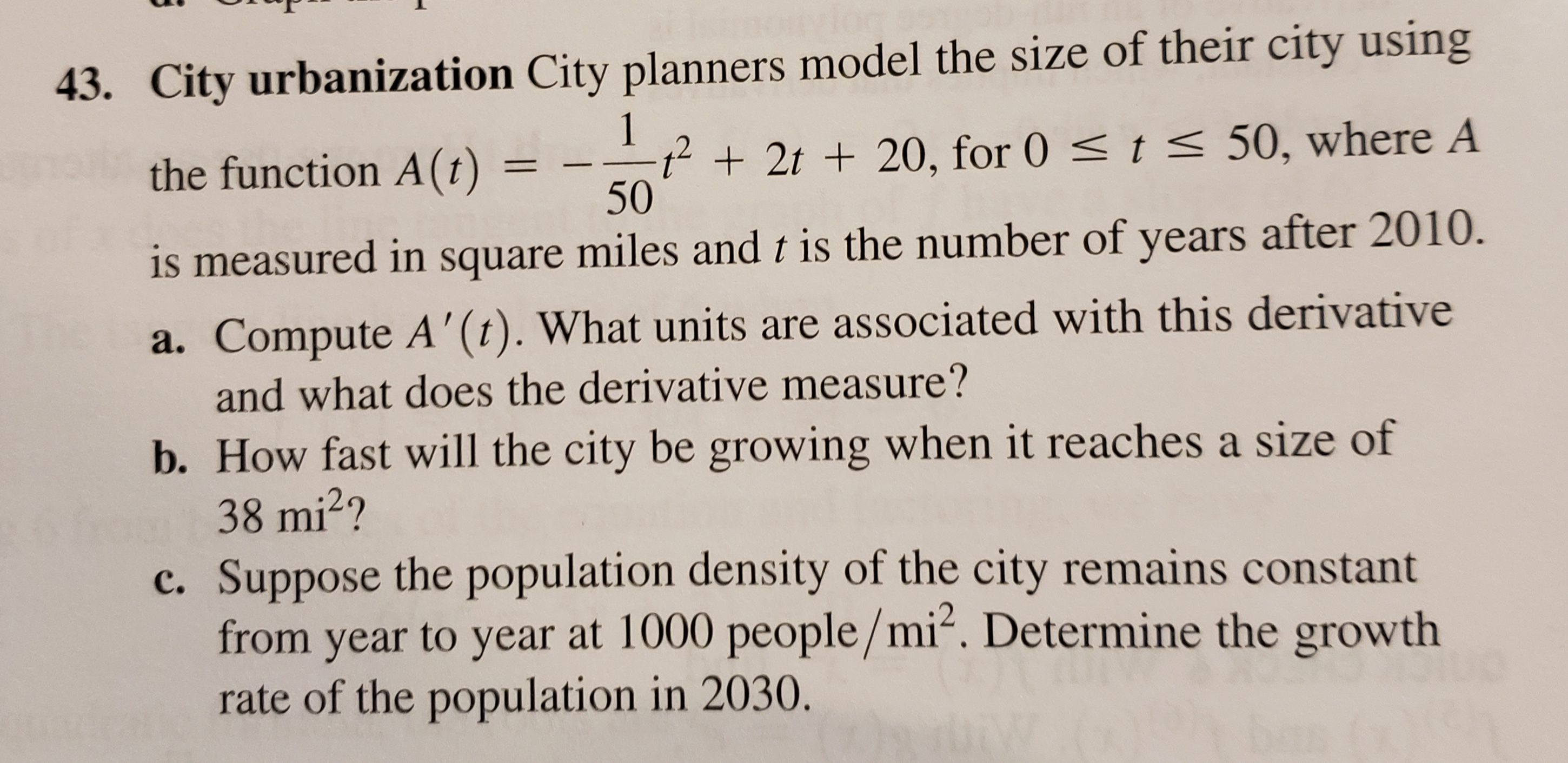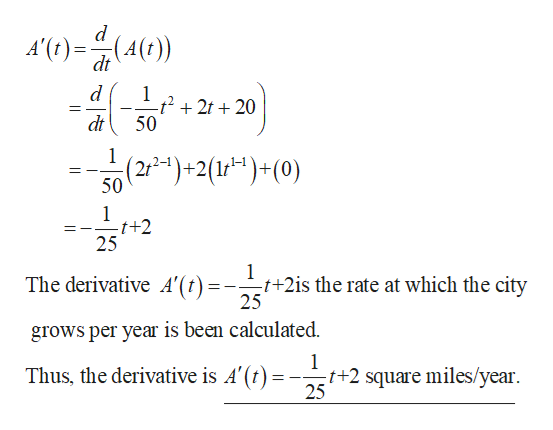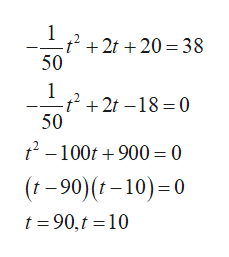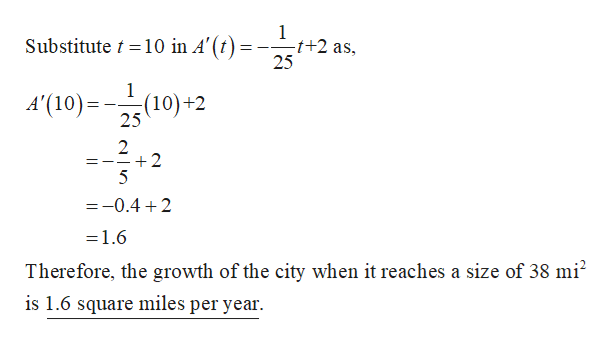43. City urbanization City planners model the size of their city using1t

Questionhelp_outlineImage Transcriptionclose43. City urbanization City planners model the size of their city using 1 t<50, where A t2t20, for 0 50 the function A(t) is measured in square miles and t is the number of years after 2010. a. Compute A'(t). What units are associated with this derivative and what does the derivative measure? b. How fast will the city be growing when it reaches a size of 38 mi2? c. Suppose the population density of the city remains constant from year to year at 1000 people/mi2. Determine the growth rate of the population in 2030. fullscreen
Step 1

a)

Obtain the derivative of A(t) as shown below.help_outlineImage TranscriptioncloseA'()4()) (4(t) dt 1 -t2 2t20 50 dt 1 (2-)+2(1 )+ (0) 50 1 t+2 25 1 -t+2is the rate at which the city 25 The derivative A'(t)=- grows per year is been calculated. 1 Thus, the derivative is A'(t) :t+2 square miles/year 25 fullscreen
Step 2

b)

Substitute A(t) = 38 in the given equation.help_outlineImage Transcriptionclose1 -t 2t 20 =38 50 1 -t 2t -18 0 50 2-100t900 0 (t-90)(-10)0 t 90,t 10 fullscreen
Step 3

The only one solution in the given do...help_outlineImage Transcriptionclose1 -t+2 as Substitute t10 in A'(t) 25 A'(10)2(10)+2 2 +2 5 =-0.4 2 =1.6 Therefore, the growth of the city when it reaches a size of 38 mi2 is 1.6 square miles per year. fullscreen

Want to see the full answer?

See Solution

Want to see this answer and more?

Our solutions are written by experts, many with advanced degrees, and available 24/7

See Solution
Tagged in

Calculus